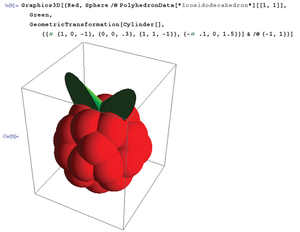Mathematica and the Wolfram language on Raspberry Pi

Graphics Tricks

Mathematica has a number of impressive functions that help you create graphical images. This article doesn't allow me the space to describe every function in detail, but the syntax is quite intuitive, and you'll find all the information you need in the Mathematica documentation.

Scientists and mathematicians use Mathematica's sophisticated 3D graphics capabilities to plot the results of complex equations and experiments, but the community also likes to play around with these features just to sharpen their skills and have a little fun. The following examples demonstrate some important graphical functions, as well as the economy and power of the Wolfram language. These examples come from either the Wolfram Mathematica one-liners competition in 2010 and 2011 or from the Raspberry Pi forum. The idea of the one-liners competition is to create the most impressive piece of code in just one line (140 characters) of code. When you are typing these bits of code, make sure to get the syntax exactly right or you might get errors or unexpected results.

The first example is Echidnahedron by Radko Kriz (Listing 1).

Listing 1

Echidnahedron

```Graphics3D[{Opacity[.8],Glow[RGBColor[1,0,0]],EdgeForm[White],Lighting -> \
None,PolyhedronData["Echidnahedron","Faces"]}]```

When you type or copy the preceding code and press Shift+Enter, you should be presented with the output in Figure 7, which is designed to look like the Mathematica "Spikey" logo in 3D.

The Hi code by Michael Croucher is a little gem found on the Raspberry Pi forums. It is effectively a 3D plot version of the "Hello world!" code (Listing 2) that produces the output shown in Figure 8.

Listing 2

3D Hi

```Plot3D[Exp[-x^2 - 0.5*y^2]*Cos[4 x] + Exp[-3 ((x + 0.5)^2 + 0.5 y^2)], {x, -3, 3}, {y, -5, 5}, \
PlotRange -> {-0.001, 0.001}, PlotPoints -> 40]```Figure 8: A really weird rendition of Hello, World.

The 3D Raspberry by Martin is included for obvious reasons. The code in Listing 3 produces a 3D version of your favorite fruit-shaped logo (Figure 9).

Listing 3

3D Raspberry

```s = Sphere; t = .3; p = PolyhedronData; Manipulate[Graphics3D@{Red, s@p[i][[1, 1]], Green, \
Rotate[Scale[s[], {t, 1, 2}, {0, 0, 3}], #, {1, 0, 0}] & /@ {-t, t}}, {i, p[]}]```Figure 9: Plotting the big raspberry in Mathematica.

GPIO Interfacing

Accessing the GPIO pins through the Wolfram language is extremely easy – potentially even easier than in other popular languages recommended for the Raspberry Pi. To start, you need to set up a basic circuit from which to interface with Mathematica. To do this, acquire the equipment in the following parts list and then follow the rest of the instructions:

• 1x red LED (Digi-Key part 67-1120-ND)
• 1x 330-ohm resistor (Digi-Key part A105936CT-ND)
• 2x male-to-female jumper wires (SparkFun part PRT-09140)

First, you need to set up the circuit, as shown in Figure 10. Make sure to include the 330-ohm resistor or you risk potentially damaging your Raspberry Pi. Also, make sure your Raspberry Pi is turned off before you attach anything to the GPIO pins; otherwise, you risk damaging both the Raspberry Pi and any device you are attaching.Figure 10: A simple circuit for experimenting with Mathematica's GPIO capabilities.

Next, boot up the Raspberry Pi and open either Mathematica or the Wolfram language command-line interface. Type in the following code, which uses the `DeviceWrite` function:

`DeviceWrite["GPIO", 14 -> 1]`

This command turns GPIO 14 (physical pin 8) to a high output and should turn on your LED.

Bear in mind that these pin numbers are not the physical pin locations, but the BCM pin designations (Broadcom SOC channels)  – as you can see on the pin diagram at the eLinux wiki page . The numbers you are looking for are all preceeded by the letters "GPIO" (General Purpose Input Output). Also, remember that if you are using a Rev 1 Raspberry Pi, some of the pins have different names from the Rev 2 Raspberry Pi board; this is also explained on the eLinux wiki page.

To turn the LED off, use the command:

`DeviceWrite["GPIO", 14 -> 0]`

With the use of a loop and the `Pause` function, you can turn the LED on and off a predetermined number of times:

```Do[
DeviceWrite["GPIO", 14->1];
Pause[0.5];
DeviceWrite["GPIO", 14->0];
Pause[0.5];
, {5}]```

This code turns the LED on, waits half a second, turns the LED off, waits half a second, then repeats the process five times.

Express-Checkout as PDF

Pages: 2

Price \$2.95
(incl. VAT)

SINGLE ISSUES

SUBSCRIPTIONS

Related content

• Exploring the Pixy sensor with Mathematica

We show how to track objects and plot their movement using the Pixy camera and the Mathematica package that's included with Raspbian.

• The Pi Wire

Things move quickly in the Raspberry Pi ecosystem. This regular column rounds up the best Raspberry Pi and open hardware news to keep you up to date on the latest projects, products, and events.

• Math, Music, and Cat Toys

Welcome to Raspberry Pi Geek – the first and only print magazine dedicated to the amazing Raspberry Pi mini-PC and the open hardware revolution. We ring in the new and old in this issue. (Actually, nothing is really very old with the Raspberry Pi, but we follow up on some previous themes, including a report on how it went for the wind-turbine-powered Raspberry Pi we described last time.)

• On the DVD